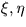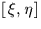Next: SLA_UE2EL - Universal to Conventional Elements
Up: SUBPROGRAM SPECIFICATIONS
Previous: SLA_TPS2C - Plate centre from and

## SLA_TPV2C - Plate centre fromand x,y,z

ACTION:
From the tangent plane coordinates of a star of known direction cosines, determine the direction cosines of the tangent point (single precision)

CALL:
CALL sla_TPV2C (XI, ETA, V, V01, V02, N)

GIVEN:

 R XI,ETA tangent plane coordinates of star (radians) V R(3) direction cosines of star

RETURNED:

 R(3) V01 direction cosines of tangent point, solution 1 V01 R(3) direction cosines of tangent point, solution 2 N I number of solutions: 0 = no solutions returned (note 2) 1 = only the first solution is useful (note 3) 2 = there are two useful solutions (note 3)

NOTES:
1.
The vector V must be of unit length or the result will be wrong.
2.
Cases where there is no solution can only arise near the poles. For example, it is clearly impossible for a star at the pole itself to have a non-zero XI value.
3.
Also near the poles, cases can arise where there are two useful solutions. The argument N indicates whether the second of the two solutions returned is useful. N=1 indicates only one useful solution, the usual case; under these circumstances, the second solution can be regarded as valid if the vector V02 is interpreted as the over-the-pole'' case.
4.
The projection is called the gnomonic projection; the Cartesian coordinatesare called standard coordinates. The latter are in units of the distance from the tangent plane to the projection point, i.e. radians near the origin.
5.
This routine is the Cartesian equivalent of the routine sla_TPS2C.Next: SLA_UE2EL - Universal to Conventional Elements
Up: SUBPROGRAM SPECIFICATIONS
Previous: SLA_TPS2C - Plate centre from and

SLALIB --- Positional Astronomy Library